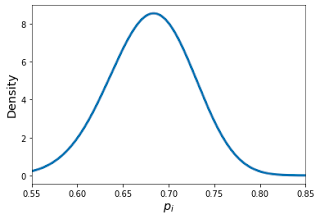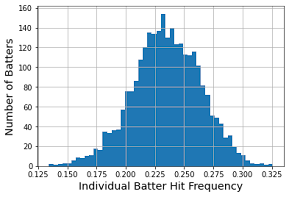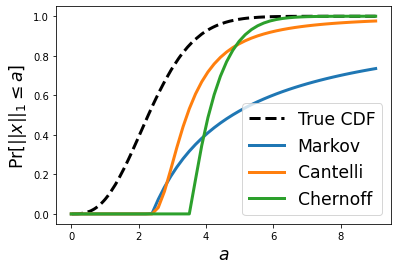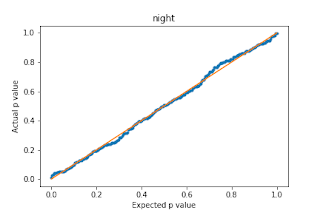## Posts

### Beat the Streak: Day NineIn this blog post, I want to talk about why getting 80% success rate in beat the streak is so challenging.  I believe I identified a mathematical reason for this, which I am going to share in this blog post.   First, lets look at some simple statistics that hint that 80% success should not be out of reach.   In the table below, we are showing the percentage of games with a hit for the most successful batters in 2011-2019. batter % Games with Hit 2011 Jacoby Ellsbury 0.821656 2012 Derek Jeter 0.812121 2013 Michael Cuddyer 0.807692 2014 Jose Altuve 0.803797 2015 Dee Gordon 0.800000 2016 Mookie Betts 0.807453 2017 Ender Inciarte 0.775641 2018 Jose Altuve 0.786207 2019 DJ LeMahieu

### Beat the Streak: Day EightIn this blog post, we will explore three factors that influence the probability of correctly selecting a player to get a hit on a given day.  These are: 1. Individual batter strength, as measured by the proportion of plate appearances that resulted in a hit. 2. Team offensive strength, as measured by the average number of plate appearances per game by the batting team.   3. The position in the batting order. We plot the distribution of these statistics over (batter, year) pairs and (team, year) pairs.  The plots below reveal that the best batters get a hit in about 30% of plate appearances, and the strongest offensive teams average 39 plate appearances per game.  The tables below show the top-performing batters and teams: batter year Josh Hamilton 2010 0.326 Trea Turner 2016 0.324 Jose Altuve 2014 0.319

### Tail Bounds on the Sum of Half Normal Random VariablesLet $x \sim \mathcal{N}(0, \sigma^2)^n$ be a vector of $n$ i.i.d normal random variables.  We wish to provide a high probability tail bound on the $L_1$ norm of $x$, that is: $$Pr[|| x ||_1 \geq a] \leq f(a)$$ Note that the term on the left is called a "tail probability" because it captures the probability mass in the tail of the distribution (i.e., values exceeding a).  This type of problem arises when we wish to claim that the probability is small for a given random variable to be large.  The tail probability above is closely related to the CDF of the random variable $|| x ||_1$: it is exactly $1 - Pr[|| x ||_1 \leq a]$.  Unfortunately, the CDF of this random variable does not appear to have a clean closed form expression, and for that reason, we wish to find a reasonably clean and tight bound on it instead.  In this blog post, we will explore, utilize, and compare the Markov inequality , the one-sided Chebyshev inequality , and the Chernoff bound to bound the de

### Open Problem: Efficiently Evaluating Subset Sums

Suppose we are given a vector of $n$ items $\begin{bmatrix} x_1 & x_2 & \dots x_n \end{bmatrix}$ and we would like to perform a number of subset sums on these items.  Specifically, we would like to compute $\sum_{i \in S} x_i$ for some collection of subsets $S \subseteq \{ 1, \dots, n \}$.   We would like to evaluate all of the summations using the minimum number of arithmetic operations (additions and subtractions). In this setting, addition and subtraction of two elements is an expensive operation (e.g., think of them as being 2D numpy arrays).  Therefore, we are willing to spend computational time and resources to find an efficient means to evaluate the subset sums.  Moreover, we may need to compute these subset sums many times, e.g., if they are computed as part of an inner loop to an iterative algorithm.  Therefore, the time spent finding the right way to evaluate the subsets sums will ultimately pay for itself. A few examples with solutions to make this problem more co

### Removing redundant constraints from a linear system of equations

Suppose you are solving an optimization problem with linear equality constraints of the form  $Ax = b$ Several off-the-shelf optimizers, including those available in CVXOPT and scipy will complain if A is not full rank.  That is, if A contains redundant constraints then optimization will likely fail.  So how can we easily deal with this issue?  The code below is something you can plug into your program for a quick fix to the issue.  Simply call A, b = reduce_row_echelon(A, b) to get an equivalent set of constraints with redundancies removed.  # Consumes a *consistent* rectangular system of equations # Produces a new compacted system of equations with linearly dependent rows removed # Note: this algorithm computes the row echelon form of the augmented matrix # This algorithm should be numerically robust def reduce_row_echelon(B, y): A = np.concatenate([B, y[:,np.newaxis]], axis=1) m,n = A.shape c = 0 for r in range(m): # find first non-zero column wh

### log-dot-exp: generalizing the log-sum-exp trick

In scientific computing applications we often have to work with very small positive numbers.  With finite precision representation for numbers (like 32 or 64 bit floats), this can be a problem when precision matters even at the smallest scales.  In these cases, it is useful to represent numbers in log space and perform computations directly in that space.  Working in log space can make a numerically unstable algorithm stable.  Suppose for example that we want to know the sum of $n$ positive real numbers: $Z = \phi_1 + \dots + \phi_n$.  In log space, we would instead work with $\theta_i = \log{(\phi_i)}$.  To perform this summation in log space, we need to evaluate the following expression: $$\log Z = \log \sum_{i} \exp{(\theta_i)}$$ When done naively, this computation can be numerically unstable, e.g., if $\theta_i$ is large then $\exp{(\theta_i)}$ may cause an overflow error.  The Log-Sum-Exp  trick provides a way to do this computation in a numerically stable manner.  In particular

### Symmetric functions and their extreme points

A symmetric function $f(x_1, \dots, x_n)$ is one that is invariant to permutations of $x$.  In the 2D case, this means $f(a,b) = f(b,a)$.  One basic example of a symmetric function is the area of a rectangle as a function of its side lengths, where $f(a,b) = a b$.  It is well known that to maximize the area of a rectangle under a constraint on the perimeter, you should set all side lengths equal, to form a square.  In this case, the maximum of this symmetric function occurs when $a=b$. While in general it is not always true that the extreme points of a symmetric function occurs when $a=b$, there are special cases when it does hold.  For example, if $f(a,b)$ is a convex (concave) function, then a global minimum (maximum) will occur when $a=b$.  Of course, the function above is not concave, but this property still holds.  So the question arises: in what other situations does this nice property hold? In this blog post, I will state a general result that I found today regarding this pr

### L2 projection onto the probability simplex with bound constraints

Projecting onto the probability simplex is a common problem that arises in frequency estimation and related tasks.  This problem can be solved efficiently with an $O(n \log{(n)})$ algorithm.  In this blog post I consider the related problem of projecting onto the probability simplex with bound constraints.  Given a vector $\mathbf{r} \in \mathbb{R}^n$, our goal is to find a vector $\mathbf{p}^*$ that solves the following optimization problem. \begin{equation*} \begin{aligned} & \underset{\mathbf{p}}{\text{minimize}} & & \frac{1}{2} \lvert \lvert \mathbf{p} - \mathbf{r} \rvert \rvert_2^2 \\ & \text{subject to} & & \mathbf{1}^T \mathbf{p} = 1 \\ & & & \mathbf{a} \leq \mathbf{p} \leq \mathbf{b} \\ \end{aligned} \end{equation*} This problem generalizes the standard probability simplex projection problem by introducing arbitrary bound constraints $\mathbf{a} \leq \mathbf{p} \leq \mathbf{b}$. Here, $\mathbf{a}, \mathbf{b} \in \mathbb{R}^n$. I

### Beyond vectorization: writing efficient numpy code

Numpy is a great library for expressing computations over vectors, matrices, and multi-dimensional arrays in python due to it’s simplicity and efficiency. In a previous blog post , I discussed fairly well-known techniques for speeding up (and cleaning up) numpy code by avoiding loops and exploiting problem structure. I showed that when you use the suggestions in that post, you can speed up your numpy code by orders of magnitude. In this blog post, I’ll show you how to get even more speed out of your numpy code on top of the improvements you get from vectorization. As a simple example to illustrate an inefficiency of numpy, consider computations of the form z = 0.2*x + 0.8*y where x and y are large numpy arrays. This computation will form intermediate arrays 0.2*x and 0.8*y, so the memory overhead can be problematic and slow down the computation. On my laptop, it takes about 1 second for arrays of size 100 million: import numpy as np def foo(x,y): return 0.2*x + 0.

### Beat the Streak: Day SevenIn this blog post, I discuss questions of the form: “Does batter X perform better at home or away?   At day or night?   Against lefties or righties? On Friday or Monday?”   What I found was a little bit surprising.   Take for example the batter Daniel Murphy.   When you look at his data from 2011 - 2017, you will see that he got a hit in 29.85% of 1424 plate appearances during day games and he got a hit in 26.97% of 2673 plate appearances during night games.   This is a pretty meaningful difference, but is it statistically significant?   In other words, could this difference be explained purely by chance?   To answer this question, we can perform a chi squared test under the null hypothesis that the true probabilities are the same.   When we do this we get a chi squared value of 3.35 and a corresponding p value of 0.067.   Thus, we can reject the null hypothesis that the true underlying probabilities are the same at the 90% confidence level.   This is pretty convincing evidence th

### Optimal Strategy for Farkle Dice

In this post, I will discuss my findings in terms of an optimal strategy for Farkle , which is a dice game of chance.  If you are unfamiliar with the game, I encourage you to skim the linked article so that you can better understand this blog post.  All of my findings are based on sound mathematical methods and a computer program was used to determine the optimal strategy.  Before I begin, let me first state the value of different dice rolls I assumed while developing the strategy. Each 1: 100 points Each 5: 50 points 3 1's: 1000 points 3 2's: 200 points ... 3 6's: 600 points 4 1's: 2000 points ... 4 6's 1200 points 5 1's 3000 points ... 5 6's 1800 points Straight 1-6 1500 points 3 Pairs (e.g., 22 33 44): 750 points Everything else is considered to be a Farkle (0 points). There are many variants to Farkle, but I like to play by this set of rules. The "game state" of Farkle roll can roughly be characterized by 2 values: the curre

### Writing Efficient Numpy CodeIn this blog post, I am going to talk about writing efficient numpy code in python. I do a good amount of numerical linear algebra for my research and personal projects, and I typically code in python with numpy (and spicy) because it is very easy to use and it is also very efficient when used correctly. Consider the following problem, which will serve as a concrete task to use as an example throughout this post. Suppose we have a (column) vector $x$ of length $n = 2^k$ and we want to compute $H_k x$ where $H_k$ is a “hierarchical” matrix with branching factor $2$, defined by \begin{align*} H_0 &= \begin{bmatrix} 1 \end{bmatrix} \\ H_{k+1} &= \begin{bmatrix} 1 & 1 \\ H_k & 0 \\ 0 & H_k \end{bmatrix} \end{align*} Where the top row is a vector of all ones and 0 denotes a matrix of zeros having the same size as $H_k$. For example, $H_2$ is a $7 \times 4$ matrix that looks like this:  \begin{bmatrix} 1 & 1 & 1 & 1 \\ 1 & 1 & 0 & 0

### Representing Graphical Model Factors in Numpy

I've been working a bit with graphical models lately for my research.  For a while I was using a library called pgmpy for their implementations of factor arithmetic and inference algorithms.  However, as my research is progressing I am needing more control than what pgmpy offers, so I decided to re-implement and extend the algorithms that I needed.  If you are in a similar situation and are finding yourself implementing your own graphical models algorithms, this post is for you. In the setting I am working in, I have a Markov random field model where there are only a small number of variables (no more than a few dozen, often much less).  However, each variable can take on a possibly large number of categorical values and the graph may contain relatively large cliques that make exact inference computationally challenging (although still possible). Suppose the model has $d$ variables and variable $i$ can take on $n_i$ possible values.  Usually a factor defined over $k$ variables